# JEE Main: How Does Bernoulli’s Principle Work

###### ByRamraj Saini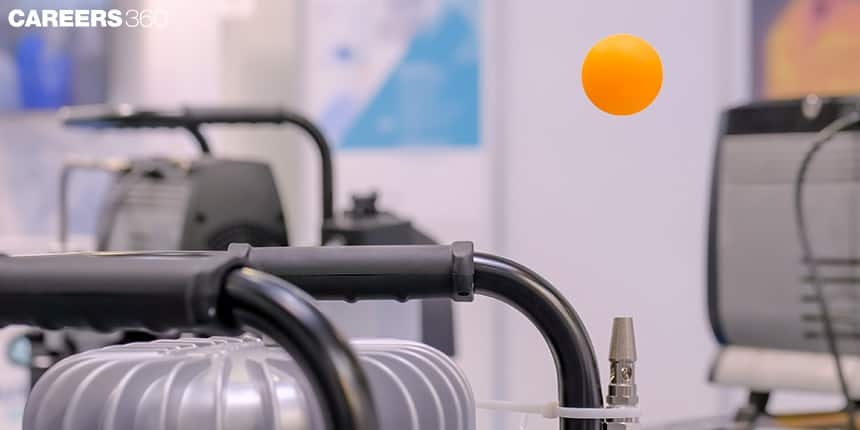###### Synopsis

Both hard work and smart study are essential to get admission to prestigious engineering institutes like the IITs, NITs and IIITs. Qualifying for the JEE main is the first step toward this. Here in this article we are going to discuss brenoulli’s principle with help of previous years questions.###### Synopsis

Both hard work and smart study are essential to get admission to prestigious engineering institutes like the IITs, NITs and IIITs. Qualifying for the JEE main is the first step toward this. Here in this article we are going to discuss brenoulli’s principle with help of previous years questions.

One of the topics in the JEE Main syllabus is Bernoulli’s principle. Questions based on finding the velocity of fluids in a bent pipe, properties of liquids, pressure difference etc. have come in the past 15 years if you look at JEE main past papers. In this article, we will decipher Bernoulli's principle, its importance for the exams and the type of questions asked from the topic in JEE Main and JEE Advanced.

A total number of 155538 candidates appeared in both papers 1 and 2 in JEE (Advanced) 2022 and a total of 40712 candidates have qualified JEE (Advanced) 2022 which makes a passing percentage of 26%.

To score well in competitive examinations, a comprehensive understanding of the topic is required which contains proper analysis of syllabus, exam pattern, previous years questions, important formulas and concepts. In this article, we will discuss Bernoulli principle and important concepts with help of previous year questions.

## What Is Bernoulli Principle?

Bernoulli principle is formulated by Daniel Bernoulli. It states that - total energy of a fluid system that contains gravitational potential energy, kinetic energy and energy associated with pressure remains constant.This principle can be formulated using the principle of conservation of energy.

## Bernoulli Principle Formula

Bernoulli principle is the relation among the pressure, kinetic energy and potential energy of fluids. The relation is given by the following formula.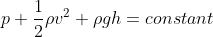Where p represents pressure exerted by the fluid, velocity of fluid is represented by V,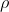is the density of fluid and h is the height of the container.

## Bernoulli Principle Derivation

Consider a pipe where an incompressible fluid is flowing and of which diameter and height are varying. The following image clearly mentions the relation between cross sectional area A, flow speed V, height y from ground and pressure P.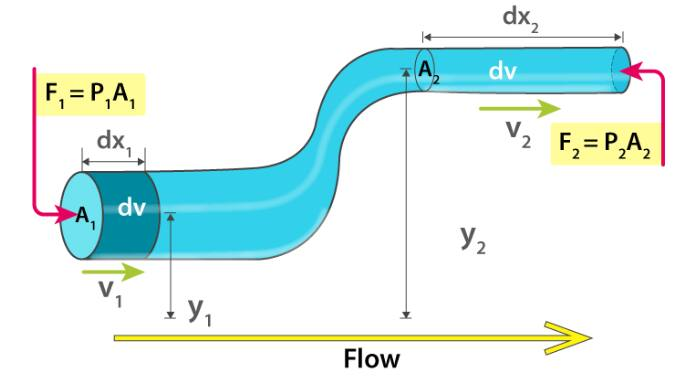Here, we take some assumptions

• Density of incompressible fluid remains constant at both points

• energy remains conserved in the system

• there is no viscous force in the fluid.

After considering the above assumptions work done on fluid is calculated below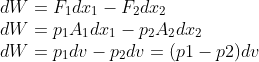We can observe that there is only one conservational force working, that is, the gravitational force. Therefore work done by fluids is equal to change in gravitational potential energy and kinetic energy of fluid.

Change in kinetic energy is given as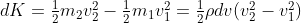The change potential energy is given as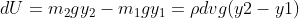Therefore the total energy equation is given as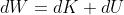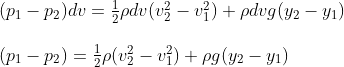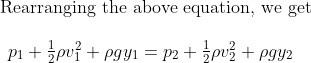This is the derivation of Bernoulli equation.

## Principle Of Continuity

According to the principle of continuity In a streamline flow when a compressible fluid is flowing then the mass of fluid passing through different cross sections are equal.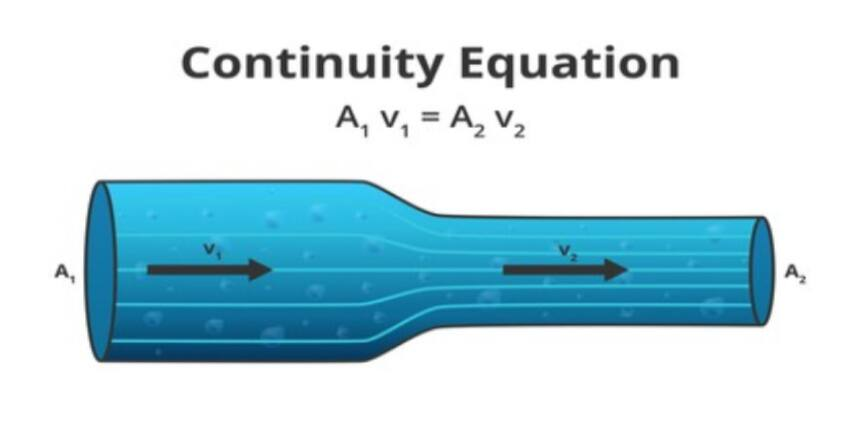We can observe that the mass of liquid remain same in the system therefore

Mass entering the system = mass leaving the system.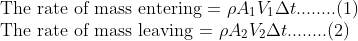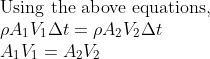This equation is known as the principle of continuity.

## Bernoulli Principle Use

There are multiple applications of Bernoulli’s principle. For example it is used in the study of unsteady flow of the ocean waves, for approximation of parameters like pressure and speed of fluids, in the working principle of Venturi meter and many more. Venturi meter is a device that is used for measuring the rate of flow of liquid through the pipes which is based on the Bernoulli principle. Another important application is in the designing of the wings of aeroplanes.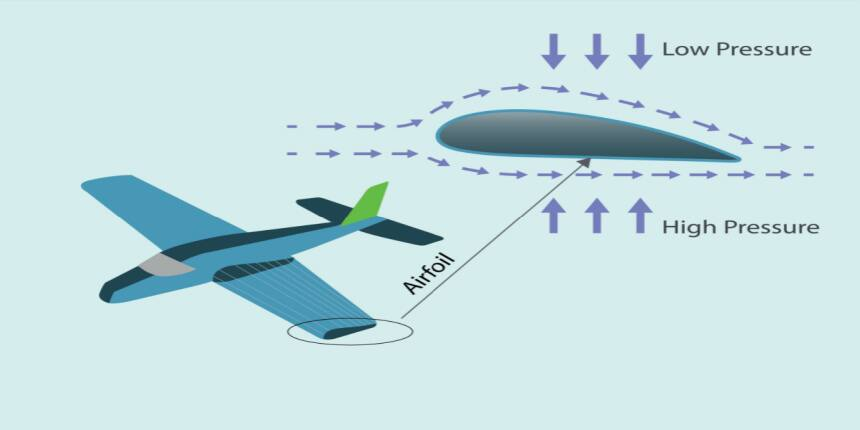## JEE Main: Previous Years Questions

Q-1 (JEE Main 2022)

An ideal fluid of density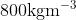, flows smoothly through a bent pipe (as shown in figure) that tapers in cross-sectional area from a to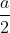. The pressure difference between the wide and narrow sections of pipe is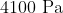. At wider section, the velocity of fluid is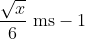for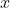= ________________.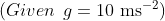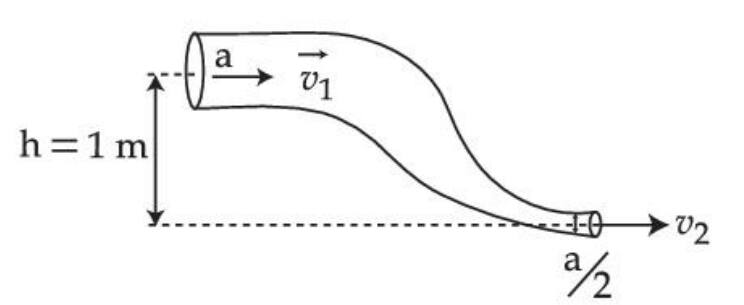Solution:

By equation of continuity ,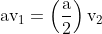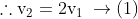By Bernoulli's theorem ,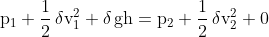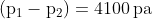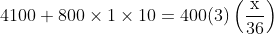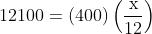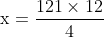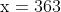Concepts Used:

• Equation of continuity

• Bernoulli principle

Q-2 (JEE Main 2022)

The area of cross-section of a large tank is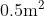. It has a narrow opening near the bottom having area of cross-section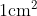. A load of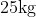is applied on the water at the top in the tank. Neglecting the speed of water in the tank, the velocity of the water, coming out of the opening at the time when the height of water level in the tank is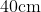above the bottom, will be ______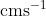. [ Take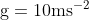]

Solution: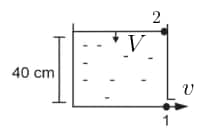Applying Bernoulli's equation at points 1 & 2 we get,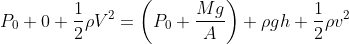Speed of free surface (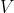) is negligible compared to the speed at the orifice (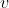)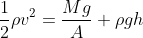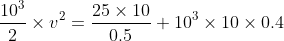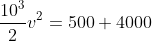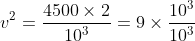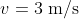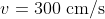Hence answer is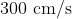Concepts Used:

• Bernoulli principle

Q-3 (JEE Main 2022)

Consider a water tank as shown in the figure. Its cross-sectional area is 0.4m2. The tank has an opening B near the bottom whose cross-section area is 1 cm2, A load of 24kg applied on the water at the top when the height of water level is 40 cm above the bottom, is the velocity of water coming out the opening B is vms-1.

The value of v, to the nearest integer, is __________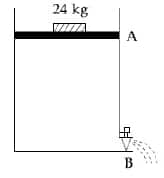Solution: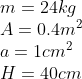Using Bernoulli's equation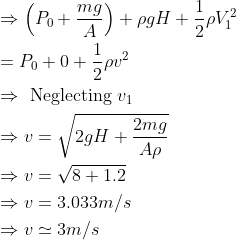Note V1 is negligible and assumed to be zero. Because the area of upper portion is very large as compare to lower hole

Concepts Used:

• Bernoulli principle

Hope you have got a bit more clarity on Bernoulli’s Principle and its application. Remember to attempt previous years questions and categorise the most important concepts as well as topics.

• Nature
• Physical sciences
• Quantity
• Physics
• Applied and interdisciplinary physics
• Physical quantities
• Chemical engineering
• Classical mechanics
• Metrology
• Phases of matter

## Careers360 helping shape your Career for a better tomorrow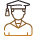#### 250M+

Students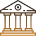#### 30,000+

Colleges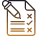#### 500+

Exams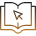E-Books